# Reactions

## Rate of Reaction

The rate of reaction is defined as the change of concentration of a reactant or product within a given time. At the start of a reaction, each reactant has the maximum concentration meaning the rate of reaction is at its fastest. As the reaction proceeds, the concentrations decrease slowing the rate of reaction.

### Factors affecting the rate of reactions

The rate of a chemical reaction is affected by:

• Temperature
• Pressure
• Concentration
• Surface area
• Use of a catalyst

The effects of these can be explained using collision theory that states that a chemical reaction can only occur when reacting molecules collide. Upon collision, the reaction only happens if the energy is greater than or equal to the activation energy.

### The effect of concentration on reaction rate

If the concentration of the reactants is increased, the rate of reaction is also increased. This is because increasing the concentration gives more molecules within the same volume, which results in a greater chance of molecules colliding. With a higher concentration, there will be more collisions with energy greater than the activation energy.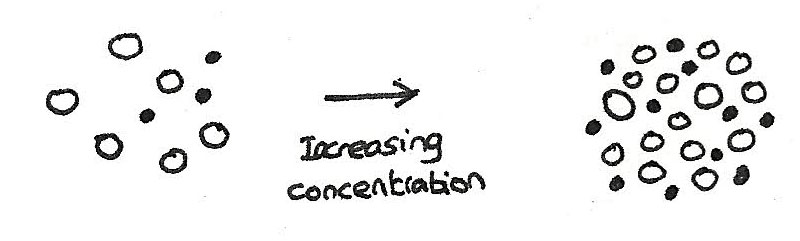### The effect of pressure on reaction rate

When the pressure of a gas is increased, the same number of molecules occupy a smaller volume. For a gaseous reaction, increasing the pressure is the same as increasing the concentration. This means that there will be more collisions with energy greater than the activation energy. More frequent collisions mean that the rate of the rate of the reaction will increase.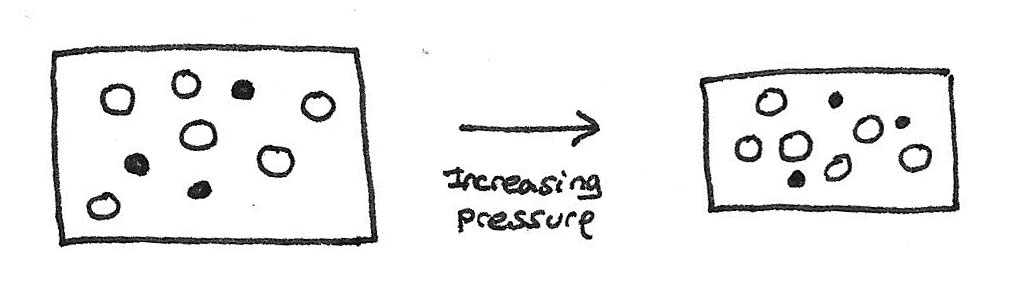## Catalysts

A catalyst increases the rate of a chemical reaction without being used up in the process. A catalyst may react to form an intermediate however before the end of the reaction it is regenerated so that it is not used up in the reaction.

A catalyst lowers the activation energy of a reaction by providing an alternative route of lower energy for the reaction to go down.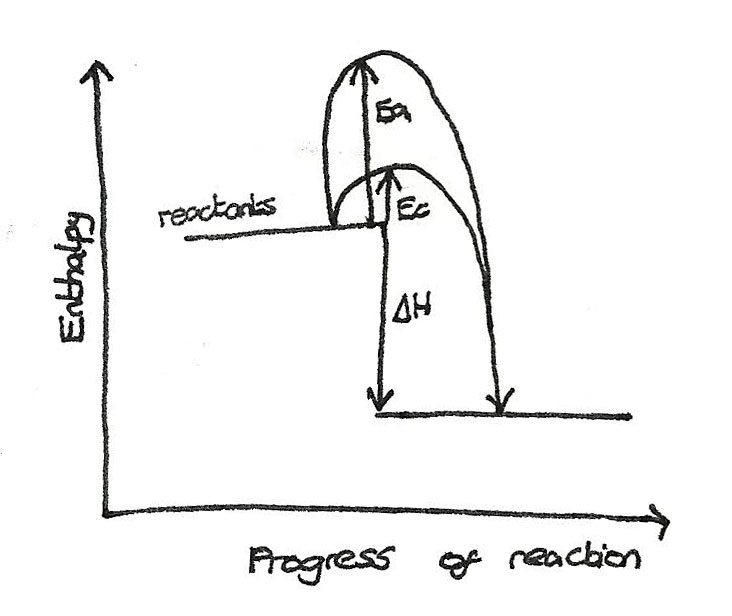In industrial processes catalysts are used to lower the activation energy, speeding up the process,which lowers the cost. Benefits of using a catalyst include:

• Less energy needed for the molecules to react. This energy is often taken from burning fossil fuels. By requiring less energy, less fuels are burned, so less carbon dioxide is released.
• Catalysts can be used to make a process more efficient by improving the percentage yield of an industrial preparation.

### Haber process

The Haber process is a process used in order to produce ammonia. The produced ammonia is used as the basis of fertiliser manufacture. Ammonia is made by reacting nitrogen and hydrogen together:

$$N_{2(g)} + 2H_{2(g)} \rightleftharpoons 2NH_{3(g)}$$

In this reaction, the triple bond in the nitrogen has to be broken, requiring high amounts of energy. When iron is used to catalyse this reaction, the nitrogen triple bond is weakened lowering the activation energy.

### Biological catalysts

Enzymes are biocatalysts which can lead to the formation of a single product, which is known as specificity. Enzymes operate under mild conditions, such as low temperatures, atmospheric pressures and at an optimum pH level.

### Enzymes in Industry

The benefits of using enzymes in industry include:

• Use of lower temperatures and pressure, saving costs and energy.
• Allow for specificity which reduces waste products and the need for separation techniques.
• Conventional catalysts can be poisonous which can cause disposal problems at the end of their lives. Enzymes are biodegradable.

## Boltzmann Distribution

A Boltzmann distribution shows the distribution of molecular energies in a gas at constant temperature. A distribution is shown below:### Features of a Distribution

• The area under the curve is equal to the number of molecules in a sample. This area is constant and does not change with conditions.
• There are no molecules in the system with zero energy as the curve starts at the origin.
• There is no maximum energy for a molecule, as the curve approaches but doesn't touch or cross the axis.
• Only the molecules with an energy greater than the activation energy, $E_{a}$ are able to react.

### Effect of temperature on the reaction rate

At higher temperatures, the kinetic energy of all the molecules increase. The distribution flattens and shifts to the right. The area and therefore the number of molecules stays the same. In the diagram below, the temperature $T_{2} > T_{1}$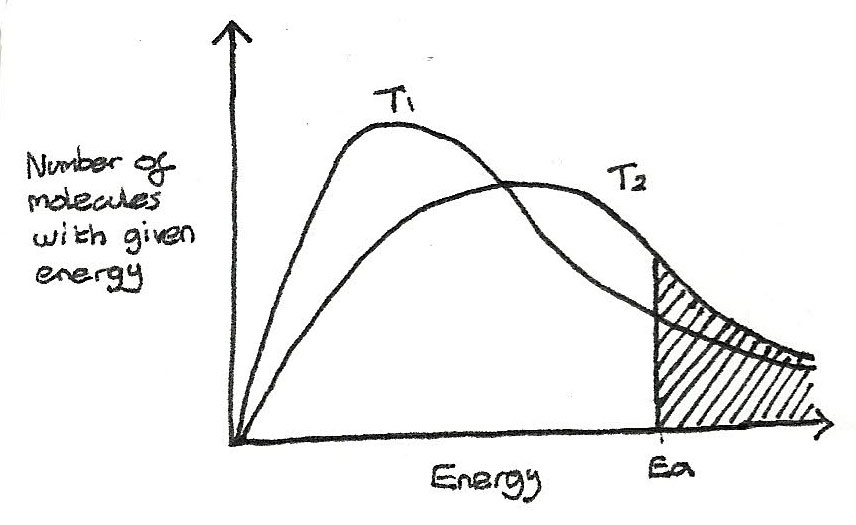As the temperature is increased, the rate of reaction increases. This is because with increased kinetic energy, more collisions are able to take place in a certain length of time and a higher proportion of molecules have energy greater than the activation energy.

### Effect of catalysts on the reaction rate

With a catalyst, the activation energy decreases so a greater proportion of molecules exceed the activation energy.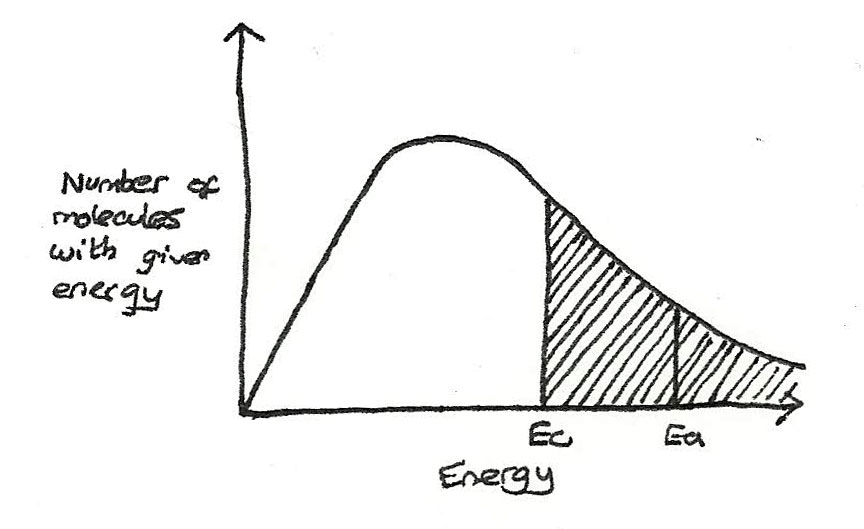## Chemical Equilibrium

Some reactions are reversible meaning they can take place in either a forwards or backwards direction. For example:

$$2SO_{2(g)} + O_{2(g)} \rightleftharpoons 2SO_{3(g)}$$

The equilibrium is indicated by the $\rightleftharpoons$ sign.

### Dynamic Equilibrium

In equilibrium, a reaction has no observable state of change. In reality, the system is dynamic where products are being formed at the same pace as reactants are being reformed. A chemical system is in dynamic equilibrium if:

• The concentrations of the products and reactants are equal.
• The rate of the forwards reaction if equal to the backwards reaction.
• The chemical system is isolated from other reactions.
• The surrounding conditions are kept constant with no extra materials added or removed.

### Position of Equilibrium

The position of equilibrium is the dominant direction the reaction is working in. This can be altered by changing the conditions of the reaction. The effect of a change can be predicted using le Chatelier's principle.

## le Chatelier's Principle

When any changes are made to the conditions of a system in equilibrium, the position of equilibrium will shift in order to oppose the change.

When the position of equilibrium is moved to the right more products are formed than reactants. To the left, more reactants are formed.

### Effect of concentration

Increasing the concentration of a reactant causes the position of equilibrium to move in the direction which decreases the concentration of the reactant. This is likewise for the products.

### Effect of pressure

Increasing the pressure of a gaseous system will make the position of equilibrium shift to the side with less moles of gas. This is because the side with the most moles is at the higher pressure. By moving the position of equilibrium to the side with less moles, the pressure is decreased.

### Worked Example

With the following equation:

$$4NH_{3(g)} + 5O_{2(g)} \rightleftharpoons 4NO_{(g)} + 6H_{2}O_{(l)}$$

What effect does an increase in total pressure have on the position of equilibrium?

This would move the position to the left, as there are only 9 moles compared with 10 on the right, which reduces the pressure of the system.

What effect will removing the nitrogen as it is produced have on the system?

This will move the position of equilibrium to the right in order to replace the removed products.

## Effect on temperature on equilibrium

How the temperature changes the position of equilibrium depends on the enthalpy change. If the forwards reaction is exothermic, increasing the temperature moves the position of equilibrium in the endothermic direction in order to reduce the temperature. If the temperature is decreased, the reaction moves in the exothermic direction in order to increase the temperature.

### Effect on a catalyst on a reaction

A catalyst does not change the position of equilibrium or the chemical composition of an equilibrium system. Instead a catalyst increases the rate of reaction of the forwards and backwards reactions equally. A catalyst increases the rate at which equilibrium is reached.

### Equilibrium is industry

Many industrial processes exist as equilibrium systems:

#### Haber Process

In the Haber Process ammonia is prepared from nitrogen and hydrogen. The reaction is shown below:

$$N_{2(g)} + 3H_{2(g)} \leftrightharpoons 2NH_{3(g)} \hspace2ex \Delta H = -92 kJ\,mol^{-1}$$

In this reaction certain conditions favour the production of ammonia:

• The forwards reaction produces fewer gas molecules, therefore by increasing the pressure more product is formed. However this requires high amounts of energy, and has safety implications.
• The forwards reaction is exothermic, therefore by reducing the temperature more product is formed. At a low temperature, the rate of reaction is decreased therefore a compromise between rate of reaction and position of equilibrium has to be made.

As a result of these disadvantages, a compromise is made between the yield and the rate of reaction:

• The temperature is kept around 400° - 500° which gives a realistic rate of reaction while still giving an acceptable yield.
• The pressure used is around 200atm which is high enough to give a high rate of reaction while not being too dangerous or using too much energy.

An iron catalyst is used which reduces the amount of energy needed for the reaction to commence.